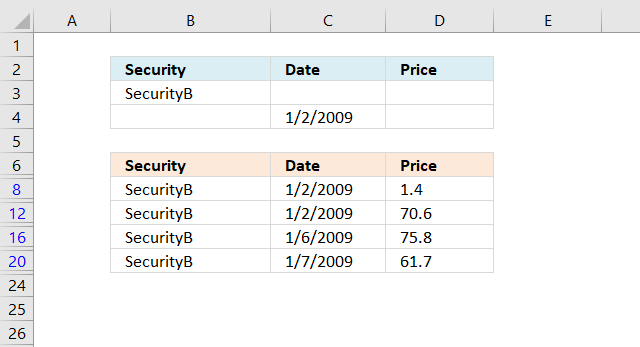Author: Oscar Cronquist Article last updated on September 24, 2021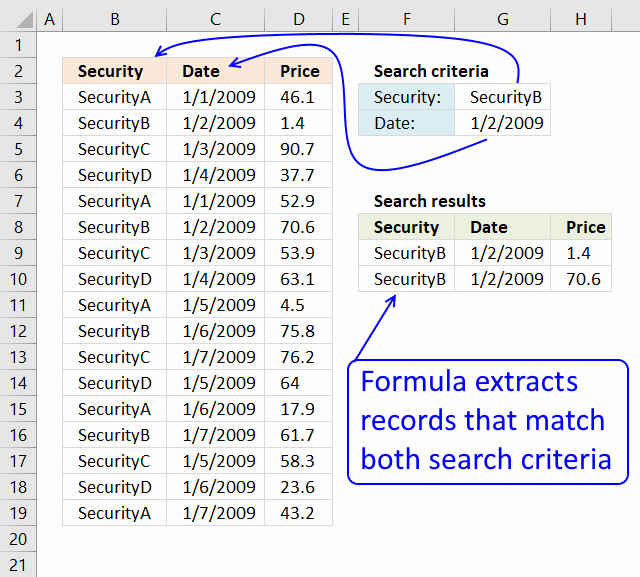This article demonstrates how to extract records/rows based on two conditions applied to two different columns, you can easily extend the formula demonstrated below to include additional criteria.

If you have a scenario where you want to apply multiple conditions on a single column then read this article: Extract all rows from a range that meet criteria in one column [Array formula]

## 1. Match two criteria and return multiple records [Array Formula]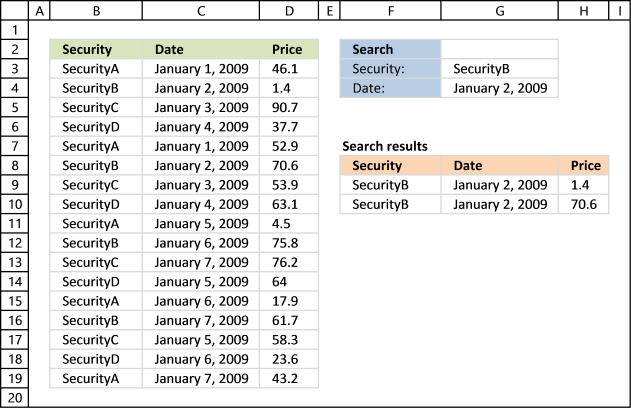The image above shows you a data set in cell range B2:D19, cell value G3 lets you match values in column B and cell G4 matches dates in column C. The formula returns matching records in cell range F9:H11 when both conditions are met.

### 1.1 Question

I have a table of 3 columns (Security name, date, price) and I have to find the price of a security at a certain date in a table that contains many securities and prices for these securities for different dates.

If I work with vlookup or Index-match I got only the first price for certain securities. So I am not able to find the price of securities that match both the name of the securities and the date.

Could you advise if there is any way to overcome this?

Array formula in F9:

=INDEX(\$B\$3:\$D\$19, SMALL(IF(COUNTIF(\$G\$3, \$B\$3:\$B\$19)*COUNTIF(\$G\$4, \$C\$3:\$C\$19), MATCH(ROW(\$B\$3:\$D\$19),ROW(\$B\$3:\$D\$19))), ROWS(\$A\$1:A1)), COLUMNS(\$A\$1:A1))

### 1.3 How to create an array formula

1. Copy (Ctrl + c) and paste (Ctrl + v) array formula into formula bar.
2. Press and hold Ctrl + Shift.
3. Press Enter once.
4. Release all keys.

Copy cell F9 and paste it to the right. Copy cell F9:H9 and paste down as far as needed.

### 1.4 Explaining excel array formula in cell range F9:H10

Use the "Evaluate Formula" tool to examine a formula in great detail. Go to tab "Formulas", press with the mouse on the "Evaluate Formula" button.

A dialog box opens, press the "Evaluate" button to see formula calculations step by step.

#### Step 1 - First condition

The COUNTIF function calculates the number of cells that is equal to a condition. We can use the COUNTIf function to match the condition to values in cell range B3:B19.

It returns an array containing as many values as there are cells in cell range B3:B19, the values can be 0 (zero) or 1. 1 indicates a match, we can use the array later on to match corresponding row numbers.

The position of a 0 (zero) or 1 in the array is important, the position matches the position in cell range B3:B19.

COUNTIF(range, criteria)

COUNTIF(\$G\$3, \$B\$3:\$B\$19)

becomes

COUNTIF("SecurityB", {"SecurityA"; "SecurityB"; "SecurityC"; "SecurityD"; "SecurityA"; "SecurityB"; "SecurityC"; "SecurityD"; "SecurityA"; "SecurityB"; "SecurityC"; "SecurityD"; "SecurityA"; "SecurityB"; "SecurityC"; "SecurityD"; "SecurityA"})

and returns {0; 1; 0; 0; 0; 1; 0; 0; 0; 1; 0; 0; 0; 1; 0; 0; 0}.

#### Step 2 - Second condition

The second condition is a date specified in cell G4, the COUNTIF function counts the condition against dates in C3:C19.

COUNTIF(\$G\$4, \$C\$3:\$C\$19)

becomes

COUNTIF(39815,{39814; 39815; 39816; 39817; 39814; 39815; 39816; 39817; 39818; 39819; 39820; 39818; 39819; 39820; 39818; 39819; 39820})

and returns {0; 1; 0; 0; 0; 1; 0; 0; 0; 0; 0; 0; 0; 0; 0; 0; 0}.

#### Step 3 - Multiply arrays - AND logic

We need both conditions to be true in order to get the correct values. The asterisk character lets you multiply the array, this is possible because both arrays have the same size.

1 * 1 = 1
1 * 0 = 0
0 * 1 = 0
0 * 0 = 0

COUNTIF(\$G\$3, \$B\$3:\$B\$19)*COUNTIF(\$G\$4, \$C\$3:\$C\$19)

becomes

{0; 1; 0; 0; 0; 1; 0; 0; 0; 1; 0; 0; 0; 1; 0; 0; 0} * {0; 1; 0; 0; 0; 1; 0; 0; 0; 0; 0; 0; 0; 0; 0; 0; 0}

and returns {0; 1; 0; 0; 0; 1; 0; 0; 0; 0; 0; 0; 0; 0; 0; 0; 0}.

#### Step 4 - Create number sequence

The ROW function calculates the row number of a cell reference. It can also return an array of row numbers if the reference is a cell range.
ROW(reference)

ROW(\$B\$3:\$D\$19)

returns {3; 4; 5; 6; 7; 8; 9; 10; 11; 12; 13; 14; 15; 16; 17; 18; 19}.

#### Step 5 - Create number sequence from 1 to n

The MATCH function returns the relative position of an item in an array or cell reference that matches a specified value in a specific order.

MATCH(lookup_value, lookup_array, [match_type])

MATCH(ROW(\$B\$3:\$D\$19),ROW(\$B\$3:\$D\$19))

becomes

MATCH({3; 4; 5; 6; 7; 8; 9; 10; 11; 12; 13; 14; 15; 16; 17; 18; 19}, {3; 4; 5; 6; 7; 8; 9; 10; 11; 12; 13; 14; 15; 16; 17; 18; 19})

and returns {1; 2; 3; 4; 5; 6; 7; 8; 9; 10; 11; 12; 13; 14; 15; 16; 17}.

This array contains row numbers containing as many numbers as there are rows in cell range \$B\$3:\$D\$19.

#### Step 6 - Replace values in array with corresponding row number

Array value 1 is replaced with the corresponding row number. 0 (zero) is replaced with nothing FALSE.

The IF function returns one value if the logical test is TRUE and another value if the logical test is FALSE.

IF(logical_test, [value_if_true], [value_if_false])

IF(COUNTIF(\$G\$3, \$B\$3:\$B\$19)*COUNTIF(\$G\$4, \$C\$3:\$C\$19), MATCH(ROW(\$B\$3:\$D\$19),ROW(\$B\$3:\$D\$19)))

becomes

IF({0; 1; 0; 0; 0; 1; 0; 0; 0; 0; 0; 0; 0; 0; 0; 0; 0}, MATCH(ROW(\$B\$3:\$D\$19),ROW(\$B\$3:\$D\$19)))

becomes

IF({0; 1; 0; 0; 0; 1; 0; 0; 0; 0; 0; 0; 0; 0; 0; 0; 0}, {1; 2; 3; 4; 5; 6; 7; 8; 9; 10; 11; 12; 13; 14; 15; 16; 17})

and returns

{FALSE; 2; FALSE; FALSE; FALSE; 6; FALSE; FALSE; FALSE; FALSE; FALSE; FALSE; FALSE; FALSE; FALSE; FALSE; FALSE}.

#### Step 7 - Extract k-th row number

The SMALL function returns the k-th smallest value from a group of numbers. It ignores text and boolean values.

SMALL(array, k)

SMALL(IF(COUNTIF(\$G\$3, \$B\$3:\$B\$19)*COUNTIF(\$G\$4, \$C\$3:\$C\$19), MATCH(ROW(\$B\$3:\$D\$19),ROW(\$B\$3:\$D\$19))), ROWS(\$A\$1:A1))

becomes

SMALL({FALSE; 2; FALSE; FALSE; FALSE; 6; FALSE; FALSE; FALSE; FALSE; FALSE; FALSE; FALSE; FALSE; FALSE; FALSE; FALSE}, ROWS(\$A\$1:A1))

The ROWS function returns the number of rows in a reference, we need the SMALL function to return a new row number in each cell, in order to do that I use the ROWS function and a reference that grows when you copy the formula and paste to cells below.

Reference \$A\$1:A1 has two parts, an absolute part \$A\$1 meaning it won't change when the formula is copied to cells below. The second part is a relative reference A1, it changes when the formula is copied.

SMALL({FALSE; 2; FALSE; FALSE; FALSE; 6; FALSE; FALSE; FALSE; FALSE; FALSE; FALSE; FALSE; FALSE; FALSE; FALSE; FALSE}, ROWS(\$A\$1:A1))

becomes

SMALL({FALSE; 2; FALSE; FALSE; FALSE; 6; FALSE; FALSE; FALSE; FALSE; FALSE; FALSE; FALSE; FALSE; FALSE; FALSE; FALSE}, 1)

and returns 2. 2 is the smallest number in the array.

#### Step 8 - Get value

The INDEX function returns a value from a cell range, you specify which value based on a row and column number.

INDEX(array[row_num][column_num], [area_num])

The COLUMNS function works just like the ROWS function except for columns instead, see the explanation above. This allows us to extract the entire row.

INDEX(\$B\$3:\$D\$19, SMALL(IF(COUNTIF(\$G\$3, \$B\$3:\$B\$19)*COUNTIF(\$G\$4, \$C\$3:\$C\$19), MATCH(ROW(\$B\$3:\$D\$19),ROW(\$B\$3:\$D\$19))), ROWS(\$A\$1:A1)), COLUMNS(\$A\$1:A1))

becomes

INDEX(\$B\$3:\$D\$19, 1, 1)

and returns "SecurityB" in cell F9.

### 1.5 Alternative array formula in F9 [Excel 2007]

=INDEX(\$B\$3:\$D\$19, SMALL(IF(COUNTIFS(\$G\$3, \$B\$3:\$B\$19, \$G\$4, \$C\$3:\$C\$19), ROW(\$B\$3:\$D\$19)-MIN(ROW(\$B\$3:\$D\$19))+1), ROW(A1)), COLUMN(A1))

How to use the INDEX function

Gets a value in a specific cell range based on a row and column number.

### Get the Excel fileSecurity1.xlsx

#### Recommended articles

The following post shows you how to filter records using a single condition:
VLOOKUP - return multiple records

## 2. Match two criteria and return multiple records [Excel 365]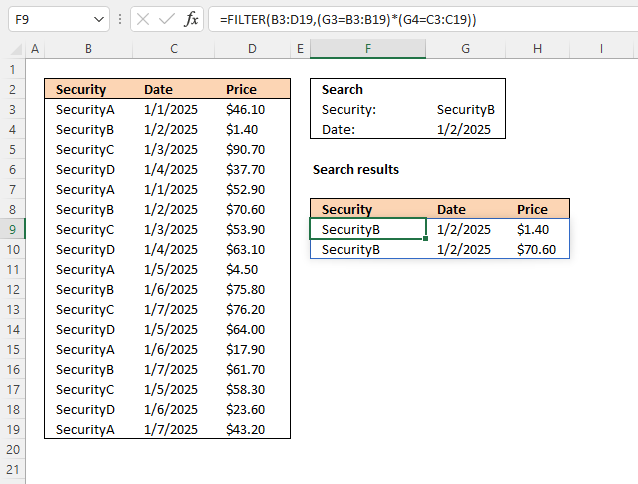Dynamic array formula in cell F9:

=FILTER(B3:D19, (G3=B3:B19)*(G4=C3:C19))

### 2.1 Explaining formula in cell F9

#### Step 1 - First condition

The equal sign is a logical operator that lets you compare value to value. In this case, a value to multiple values, the result is an array containing boolean values True or False.

G3=B3:B19

becomes

"SecurityB"={"SecurityA"; "SecurityB"; "SecurityC"; "SecurityD"; "SecurityA"; "SecurityB"; "SecurityC"; "SecurityD"; "SecurityA"; "SecurityB"; "SecurityC"; "SecurityD"; "SecurityA"; "SecurityB"; "SecurityC"; "SecurityD"; "SecurityA"}

and returns

{FALSE; TRUE; FALSE; FALSE; FALSE; TRUE; FALSE; FALSE; FALSE; TRUE; FALSE; FALSE; FALSE; TRUE; FALSE; FALSE; FALSE}.

#### Step 2 - Second condition

The second condition identifies cells in cell range C3:C19 containing the date specified in cell G4.

G4=C3:C19

becomes

45659={45658; 45659; 45660; 45661; 45658; 45659; 45660; 45661; 45662; 45663; 45664; 45662; 45663; 45664; 45662; 45663; 45664}

and returns

{FALSE; TRUE; FALSE; FALSE; FALSE; TRUE; FALSE; FALSE; FALSE; FALSE; FALSE; FALSE; FALSE; FALSE; FALSE; FALSE; FALSE}.

#### Step 3 - AND logic

Both conditions must be met, the asterisk character allows you to multiply the arrays meaning AND logic is applied.

TRUE * TRUE = TRUE (1)
TRUE * FALSE = FALSE (0)
FALSE * TRUE = FALSE (0)
FALSE * FALSE = FALSE (0)

When boolean values are multiplied their numerical equivalents are returned. TRUE = 1 and FALSE = 0 (zero).

(G3=B3:B19)*(G4=C3:C19)

becomes

{FALSE; TRUE; FALSE; FALSE; FALSE; TRUE; FALSE; FALSE; FALSE; TRUE; FALSE; FALSE; FALSE; TRUE; FALSE; FALSE; FALSE} * {FALSE; TRUE; FALSE; FALSE; FALSE; TRUE; FALSE; FALSE; FALSE; FALSE; FALSE; FALSE; FALSE; FALSE; FALSE; FALSE; FALSE}

and returns

{0; 1; 0; 0; 0; 1; 0; 0; 0; 0; 0; 0; 0; 0; 0; 0; 0}.

#### Step 4 - Extract records

The FILTER function lets you extract values/rows based on a condition or criteria. It is in the Lookup and reference category and is only available to Excel 365 subscribers.

FILTER(array, include, [if_empty])

FILTER(B3:D19, (G3=B3:B19)*(G4=C3:C19))

becomes

FILTER(B3:D19, {0; 1; 0; 0; 0; 1; 0; 0; 0; 0; 0; 0; 0; 0; 0; 0; 0})

and returns

{"SecurityB", 45659, 1.4; "SecurityB", 45659, 70.6}.

### Get the Excel fileSecurity4.xlsx

## 3. Match two criteria and return multiple records [Excel defined Table]

1. Select the range2. Press with left mouse button on "Insert" tab
3. Press with left mouse button on "Table"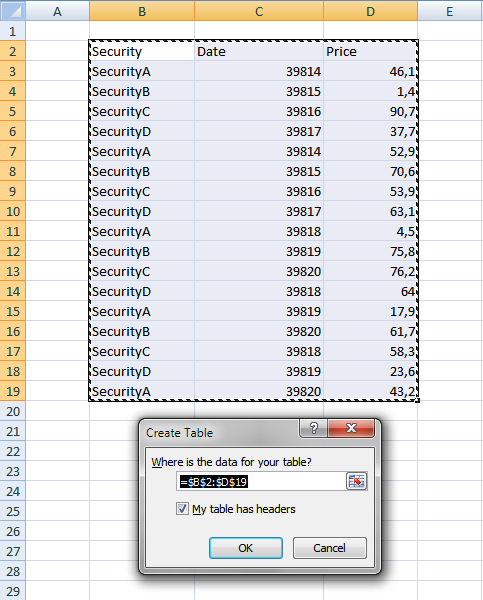4. Press with left mouse button on OK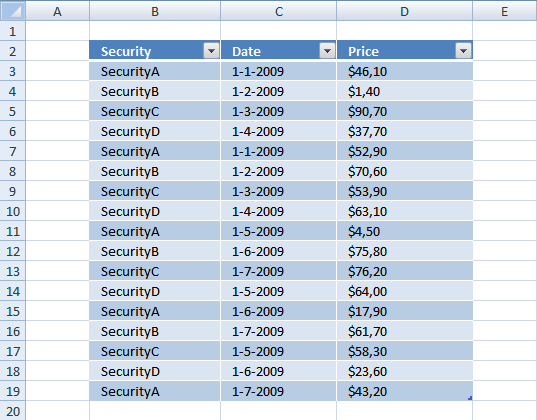1. Press with left mouse button on black arrow next to header "Security".
2. Select the items you want to filter.
3. Press with left mouse button on black arrow next to header "Date".
4. Make sure only 1-2-2009 is selected.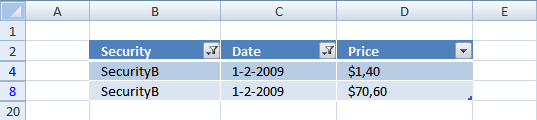The image above shows both conditions applied to the Excel Table.

## 4. Match two criteria and return multiple records [Advanced Filter]The image above demonstrates a filter applied to a data set using Excel's Advanced Filter feature. Here is how to create that filter:

1. Copy headers and paste to cells below or above the dataset.
Note, the filter values may become hidden if you place them next to the dataset.
2. Type the conditions below each header accordingly.
3. Select the dataset.
4. Go to tab "Data" on the ribbon.
5. Press with left mouse button on "Advanced" button, a dialog box appears.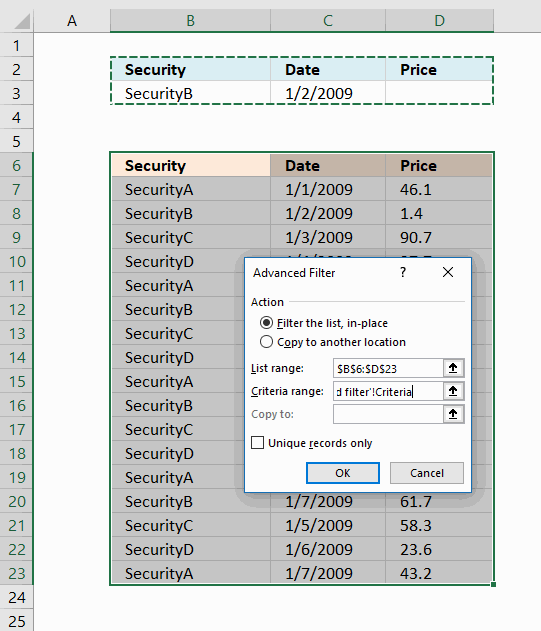6. Press with left mouse button on radio button "Filter the list, in place".
7. Press with left mouse button on "Criteria range:" field and select cell range B2:D3, see image above.
8. Press with left mouse button on OK button.The image above shows records filtered on items based on condition in B3 and dates based on condition in C3. If both conditions match on the same row the record/row appears in the filtered list.

Put the conditions on a row each in order to apply OR-logic instead of AND-logic between conditions, see image below.